# Anatolii V. Zhuchok – The least dimonoid congruence on the free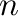-nilpotent-dimonoid

Luhansk Taras Shevchenko National University (Starobilsk, Ukraine)
The-dimonoids  are a generalization of dimonoids  while every 0-dialgebra with associative operations  is a linear analog of a-dimonoid. Free-nilpotent-dimonoids were constructed in .

Letbe an arbitrary nonempty set, and letbe an arbitrary word over. For every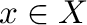, the number of occurrences of the element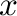inis denoted by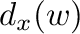. Ifis a congruence on a-dimonoid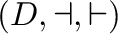such that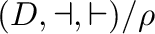is a dimonoid, we say thatis a dimonoid congruence. Ifis a congruence on a-dimonoidsuch that operations ofcoincide, we say thatis a semigroup congruence.

Theorem. Let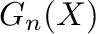be the free-nilpotent-dimonoid,.

(i) Define a relation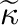onby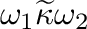if and only if one of the following conditions holds:

(1)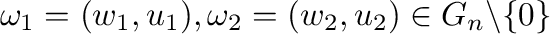and,;

(2)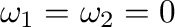.

Thenis the least dimonoid congruence on.

(ii) Define a relationonby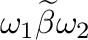if and only if one of the following conditions holds:

(1)and;

(2).

Thenis the least semigroup congruence on.

References


Loday, J.-L.: Dialgebras. In: Dialgebras and related operads: Lect. Notes Math., vol. 1763, Berlin: Springer-Verlag, 7–66 (2001)

Movsisyan, Y., Davidov, S., Safaryan, M.: Construction of free-dimonoids. Algebra Discrete Math. 18, no. 1, 138–148 (2014)

Pozhidaev, A.P.: 0-dialgebras with bar-unity and nonassociative Rota-Baxter algebras. Sib. Math. J. 50, no. 6, 1070–1080 (2009)

Zhuchok, Yul. V.: On one class of algebras. Algebra Discrete Math. 18, no. 2, 306–320 (2014)